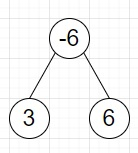# Program to find most frequent subtree sum of a binary tree in Python

Suppose we have a binary tree, we have to find the most frequent subtree sum. The subtree sum of a node is actually the sum of all values under a node, including the node itself.

So, if the input is likethen the output will be 3 as it occurs twice − once as the left leaf, and once as the sum of 3 - 6 + 6.

To solve this, we will follow these steps −

• count := an empty map
• Define a function getSum() . This will take node
• if node is null, then
• return 0
• mySum := getSum(left of node) + getSum(right of node) + value of node
• count[mySum] := count[mySum] + 1
• return mySum
• From the main method do the following
• getSum(root)

Let us see the following implementation to get better understanding −

## Example

Live Demo

from collections import defaultdict
class TreeNode:
def __init__(self, data, left = None, right = None):
self.val = data
self.left = left
self.right = right
class Solution:
def solve(self, root):
count = defaultdict(int)
def getSum(node):
if not node:
return 0
mySum = getSum(node.left) + getSum(node.right) + node.val
count[mySum] += 1
return mySum
getSum(root)
return max(count, key=count.get)
ob = Solution()
root = TreeNode(-6)
root.left = TreeNode(3)
root.right = TreeNode(6) print(ob.solve(root))

## Input

root = TreeNode(-6)
root.left = TreeNode(3)
root.right = TreeNode(6)

## Output

3